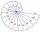# Square root + cube - math problems

#### Number of problems found: 47

• CubesOne cube is an inscribed sphere and the other one described. Calculate the difference of volumes of cubes, if the difference of surfaces in 257 mm2.
• HoleIn the center of the cube with edge 14 cm we will drill cylinder shape hole. Volume of the hole must be 27% of the cube. What drill diameter should be chosen?
• ConeCircular cone with height h = 29 dm and base radius r = 3 dm slice plane parallel to the base. Calculate the distance of the cone vertex from this plane, if solids have the same volume.
• PowerNumber left(sqrt(14 * sqrt[ 4 ] (14)) right) 17 can be written in the form 14^x. Find the value of x.
• RootUse law of square roots roots: cbrt (sqrt (sqrt (6))) = sqrt[n] (6)
• Cube wallThe surface of the first cube wall is 64 m2. The second cube area is 40% of the surface of the first cube. Determine the length of the edge of the second cube (x).
• Cube diagonalDetermine the length of the cube diagonal with edge 37 mm.

We apologize, but in this category are not a lot of examples.
Do you have an exciting math question or word problem that you can't solve? Ask a question or post a math problem, and we can try to solve it.

We will send a solution to your e-mail address. Solved examples are also published here. Please enter the e-mail correctly and check whether you don't have a full mailbox.

Square root - math problems. Cube Problems.